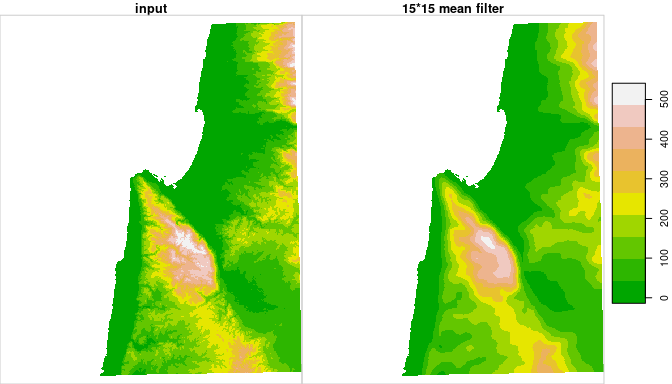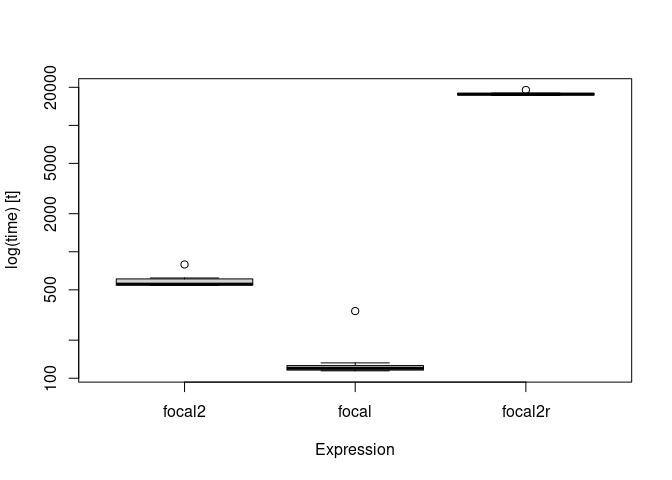R package starsExtra provides several miscellaneous functions for working with stars objects, mainly single-band rasters. Currently includes functions for:

• Focal filtering
• Detrending of Digital Elevation Models
• Calculating flow length
• Calculating the Convergence Index
• Calculating topographic aspect and slope

## Installation

CRAN version:

install.packages("starsExtra")

GitHub version:

install.packages("remotes")
remotes::install_github("michaeldorman/starsExtra")

## Usage

Once installed, the library can be loaded as follows.

library(starsExtra)

## Documentation

The complete documentation can be found at https://michaeldorman.github.io/starsExtra/.

## Example

The following code applied a 15*15 mean focal filter on a 533*627 stars Digital Elevation Model (DEM):

data(carmel)
carmel_mean15 = focal2(
x = carmel,             # Input 'stars' raster
w = matrix(1, 15, 15),  # Weights
fun = "mean",           # Aggregation function
na.rm = TRUE,           # 'NA' in neighborhood are removed
mask = TRUE             # Areas that were 'NA' in 'x' are masked from result
)

The calculation takes: 0.5625446 secs.

The original DEM and the filtered DEM can be combined and plotted with the following expressions:

r = c(carmel, carmel_mean15, along = 3)
r = st_set_dimensions(r, 3, values = c("input", "15*15 mean filter"))
plot(r, breaks = "equal", col = terrain.colors(10), key.pos = 4)## Timing

The following code section compares the calculation time of focal2 in the above example with raster::focal (both using C/C++) and the reference method focal2r (using R code only).

library(microbenchmark)
library(starsExtra)
library(raster)

data(carmel)
carmelr = as(carmel, "Raster")

res = microbenchmark(
focal2 = focal2(carmel, w = matrix(1, 15, 15), fun = "mean", na.rm = FALSE),
focal = focal(carmelr, w = matrix(1, 15, 15), fun = mean, na.rm = FALSE),
focal2r = focal2r(carmel, w = matrix(1, 15, 15), mean),
times = 10
)
res
#> Unit: milliseconds
#>     expr        min         lq       mean     median         uq        max neval
#>   focal2   542.3506   546.0164   587.9224   554.3557   609.8761   793.5663    10
#>    focal   114.3561   115.9764   142.2213   119.7932   125.9327   339.7064    10
#>  focal2r 17236.5407 17367.5100 17734.2765 17634.1378 17902.5663 19048.0060    10
boxplot(res)